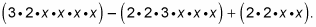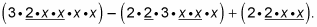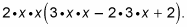##### Pre-Calculus: 1001 Practice Problems For Dummies (+ Free Online Practice)No matter how many terms a polynomial has, you always want to check for a greatest common factor (GCF) first. If the polynomial has a GCF, factoring the rest of the polynomial is much easier because once you factor out the GCF, the remaining terms will be less cumbersome. If the GCF includes a variable, your job becomes even easier.

When solving for x in a polynomial equation, if you forget to factor out the GCF, you may miss a solution, and that could mix you up in more ways than one! Without that solution, you could end up with an incorrect graph for your polynomial. And then all your work would be for nothing!

To factor the polynomial 6x4 – 12x3 + 4x2, for example, follow these steps:

1. Break down every term into prime factors.

This step expands the original expression to2. Look for factors that appear in every single term to determine the GCF.

In this example, you can see one 2 and two x’s in every term:The GCF here is 2x2.

3. Factor the GCF out from every term in front of parentheses and group the remnants inside the parentheses.

You now have4. Multiply each term to simplify.

The simplified form of the expression you find in Step 3 is 2x2(3x2 – 6x + 2).

To see if you factored correctly, distribute the GCF and see if you obtain your original polynomial. If you multiply the 2x2 inside the parentheses, you get 6x4 – 12x3 + 4x2. You can now say with confidence that 2x2 is the GCF.